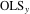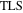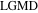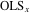# Volume 16

## Development of Simulation Models of Respiratory Tracking and Synchronizing for Radiotherapy »NBCDFPDF

Published December 18, 2014

Remarkable advances have been made in radiotherapy for cancer. Tumors inside the body that move with respiration can now be tracked during irradiation or can be irradiated at a specific phase of respiration . Since radiation of these wavelengths is invisible to the human eye, it is not possible to see whether a tumor that is moving during respiration is being irradiated accurately. Therefore, simulations that use the actual respiration wave to show the tumor being irradiated are useful for training and to explain the procedure to patients. Read More »

## Evaluation of Gaussian Molecular Integrals »NBCDFPDF

### III. Nuclear-Electron Attraction Integrals

Published December 8, 2014

This article carries out the evaluation of nuclear-electron attraction energy integrals using Gaussian-type functions with arbitrary Cartesian angular values. As an example, we calculate the corresponding matrix for the water molecule in the STO-3G basis set. Read More »

## On Bürmann’s Theorem and Its Application to Problems of Linear and Nonlinear Heat Transfer and Diffusion »NBCDFPDF

### Expanding a Function in Powers of Its Derivative

Published November 24, 2014

This article presents a compact analytic approximation to the solution of a nonlinear partial differential equation of the diffusion type by using Bürmann’s theorem. Expanding an analytic function in powers of its derivative is shown to be a useful approach for solutions satisfying an integral relation, such as the error function and the heat integral for nonlinear heat transfer. Based on this approach, series expansions for solutions of nonlinear equations are constructed. The convergence of a Bürmann series can be enhanced by introducing basis functions depending on an additional parameter, which is determined by the boundary conditions. A nonlinear example, illustrating this enhancement, is embedded into a comprehensive presentation of Bürmann’s theorem. Besides a recursive scheme for elementary cases, a fast algorithm for multivalued Bürmann expansions and inverse functions is developed using integer partitions. The present approach facilitates the search for expansions of analytic functions superior to commonly used Taylor series and shows how to apply these expansions to nonlinear PDEs of the diffusion type. Read More »

## Three Ways to Solve Domino Grids »NBCDFPDF

Published October 17, 2014

This article develops and compares three methods for solving domino grid puzzles: a “human-type” algorithm, a brute-force method, and a scheme using a generalized odometer. Read More »

## Representing Families of Cellular Automata Rules »NBCDFPDF

Published August 25, 2014

This article introduces the notion of a representation of cellular automata rules based on a template. This enhances the standard representation based on a rule table, in that it refers to families of cellular automata, instead of a rule alone. The key for obtaining the templates is the role of the built-in equation-solving capabilities of Mathematica. Operations applicable to the templates are defined, and examples of their use are given in the context of finding representations for rule sets that share the properties of maximum internal symmetry or number conservation. The perspectives for using templates in further contexts are also discussed and current limitations are addressed. Read More »

## Complex System Reliability »NBCDFPDF

### A Graph Theory Approach

Published July 16, 2014

We demonstrate a method of generating an exact analytical expression for the reliability of a complex system using a directed acyclic graph to represent the system’s reliability block diagram. Additionally, we show how statistical information stored in a reliability block diagram can be used to transform an analytical expression into a time-dependent function for system reliability. Read More »

## Canonical Correlation Analysis »NBCDFPDF

Published June 23, 2014

This article performs a canonical correlation analysis on financial data of country-specific Exchange Traded Funds (ETFs) to analyze the relationship between stock markets in developed and developing countries. We conclude, using Bartlett’s statistic, that there is a significant relationship between these two datasets. Read More »

## The Arbelos »NBCDFPDF

### Incircle, Radical Circle, Radical Axis, Twins, Generalizations, and Proofs without Words

Published May 20, 2014

This article systematically verifies a series of properties of an ancient figure called the arbelos. It includes some new discoveries and extensions contributed by the author. Read More »

## Fitting Data with Different Error Models »NBCDFPDF

Published April 30, 2014

A maximum likelihood estimator has been applied to find regression parameters of a straight line in case of different error models. Assuming Gaussian-type noise for the measurement errors, explicit results for the parameters can be given employing Mathematica. In the case of the ordinary least squares (), total least squares (), and least geometric mean deviation () approaches, as well as the error model of combining ordinary least squares (and) in the Pareto sense, simple formulas are given to compute the parameters via a reduced Gröbner basis. Numerical examples illustrate the methods, and the results are checked via direct global minimization of the residuals. Read More »

## Using Reduce to Compute Nash Equilibria »NBCDFPDF

### Classroom Tools for Game Theory

Published March 20, 2014

The Karush-Kuhn-Tucker equations (under suitable conditions) provide necessary and sufficient conditions for the solution of the problem of maximizing (minimizing) a concave (convex) function. This article corrects the program in , which computes the solution of Karush-Kuhn-Tucker equations. Our main goal, however, is to provide a program to compute the set of all Nash equilibria of a bimatrix game. The program works well for “small” games (i.e. 4×4 or smaller games); thus, in particular, it is suitable for constructing classroom examples and as an additional tool to empower students in classes using game theory. Read More »